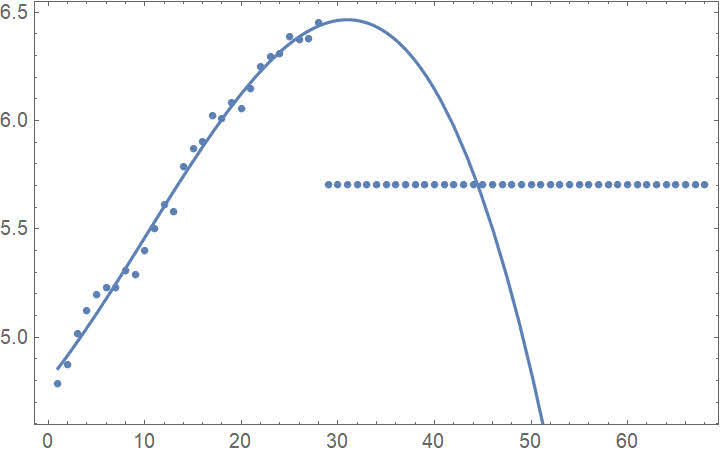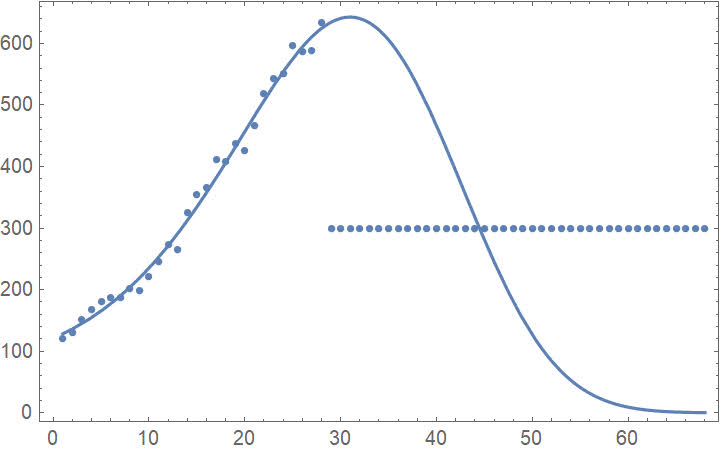## Wednesday, October 21, 2020 ...//### Optimistic cubic fits for log Covid hospitalizations

If you're worried about you and SARS-CoV-2, simply use a mouthwash (as simple as Listerine) for 30 seconds. It will inactivate over 99.9% of the viruses, a Penn State paper showed. A summary is here. Note that it is a good idea to do an experiment whenever Donald Trump invents a potentially great idea such as the drinking of bleach!Sorry, sufferers from the Trump Derangement Syndrome but the Donald was basically and preciously right on this one, too.

OK, now when America was made great again and Covid-19 has been turned into a purely academic problem, let me show you a simple interpolation that makes some sense as a method to forecast numbers that tend to exponentially grow or decay. An infectious disease has the precise exponential growth and/or the precise exponential decrease as the most typical behavior, a zeroth-order approximation. On top of that, the exponential speedup is universally slowing down, the doubling times are getting lower, before the growth switches to a decrease. This optimistic evolution is unavoidable because every dramatically growing disease – whose graph resembles the Tesla stock or any other Ponzi scheme – is ultimately running out of victims, just like the Ponzi schemes do.

Here is a simple example of a cubic fit you may play with.

I will discuss the number of Covid patients in a serious condition in the Czech hospitals. The latest number is 634 and we won't run out of the ICU beds, let alone anything else, calm down.

Fine. The ICUs are still ready almost for a tripling of the serious cases. It has virtually no chance of occurring, as we will also see. The other gadgets related to the Covid patients in hospital are even safer.

But a funny thing is that a month ago, the evolution looked like a clearly accelerating, convex, function. In the recent 2 weeks, the evolution of the serious cases is approximately linear and starts to be slightly concave (a quadratic fit from the recent 14 daily data has a negative quadratic coefficient). It means that the second derivative has decreased – the third derivative of the number of serious cases as a function of time is negative e.g. in the recent 28 days. OK, let's copy the latest 28 numbers of the Czech serious cases:
a = {120, 131, 151, 168, 181, 187, 187, 202, 198, 221, 245, 274, 265, 326, 354, 366, 412, 408, 438, 426, 467, 518, 543, 551, 596, 587, 589, 634};
le = Length[a]
ListLinePlot[a]
a2 = Table[i, {i, 1, le}];
a3exp = Transpose[{a2, a}];
a3 = Transpose[{a2, Log[a]}];
a4x = Table[i, {i, 1, le + extradays}];
along = a~Join~Table[300, {i, 1, extradays}];
a4exp = Transpose[{a4x, along}];
a4 = Transpose[{a4x, Log[along]}];
Excellent. I created various rectangular arrays which are 28 or 28+40 days long (we will only predict 40 days in advance). Here is the Wolfram Mathematica command to calculate the nonlinear model:
nlm = NonlinearModelFit[a3, c1 + c2*x + c3*x^2 + c4*x^3, {c1, c2, c3, c4}, x]
Normal[nlm]
Show[ListPlot[a3], Plot[nlm[x], {x, 1, le}], Frame -> True]
Show[ListPlot[a3exp], Plot[Exp[nlm[x]], {x, 1, le}], Frame -> True]
Here are similar plotting commands with the extra 40 days:
Show[ListPlot[a4], Plot[nlm[x], {x, 1, le + extradays}], Frame -> True]
Show[ListPlot[a4exp], Plot[Exp[nlm[x]], {x, 1, le + extradays}], Frame -> True]
OK, the Ansatz for the function (the number of serious patients in hospitals) was "exp of a cubic function of time". The exponential is natural because we want to produce non-negative numbers of patients at all times (and the exponential growth is the "norm", anyway). The cubic coefficient corresponds to the change of the "exponential rate", a term that is responsible for the gradual transition from the accelerated growth to the exponential decay. OK, the natural logarithms of the projections look like this:while the plot with the normal absolute numbers look like this:Great. The horizontal dots in the middle indicate the period in which the data is predicted. You may see that this Ansatz predicts the peak of the serious hospitalized patients in 4 days or so, around 650 (plus minus noise), not very far from the current numbers, and the subsequent decrease is predicted to be dramatic. Well, it's a basic trait of our fit that the cubic term dominates in the far future which means that the number of hospitalizations is predicted to drop "faster than exponentially". Clearly, this is an optimistic scenario of a sort because the rate at which the decay accelerates cannot be sustained. On top of that, there will always be some longer-term patients in the hospital, and so on.

OK, this code also estimates the number of deaths (daily deaths are expected to be proportional to the current serious hospitalizations, the coefficient ends up being 0.106, and the code is below):
deaths = Reverse[{97, 71, 71, 71, 52, 60, 48, 64, 42, 50, 59, 25, 45, 31, 27, 32, 13, 21, 27, 15, 20, 11, 16, 16, 10, 10, 21, 10}]
Length[deaths]
ListLinePlot[deaths]
coef = Total[deaths]/Total[a] + 0.
expectedDeaths = Table[Exp[nlm[day]]*coef, {day, 29, le + extradays}];
Round[expectedDeaths]
Total[expectedDeaths]
Excellent, with this Ansatz, the deaths for coming days would be rounded to
{67, 68, 68, 68, 67, 66, 64, 62, 60, 57, 53, 50, 46, 42, 38, 34, 30, 26, 23, 19, 16, 13, 11, 9, 7, 6, 4, 3, 3, 2, 1, 1, 1, 0, 0, 0, 0, 0, 0, 0}
whose sum is about 1085. The deaths in recent days are slightly underestimated by the fit above, perhaps by 10%, but it's still true that slightly above 1000 extra deaths would be predicted for the coming 40 days or so, after which the problems would be over. If true, the total Czech deaths with Covid would be below 3,000.

Without the exponentiation and by eyeballing and a more instinctive estimate, I got a slightly later peak, almost 2 weeks from now, and the total number of deaths around 4200. Maybe even that estimate could be slightly optimistic but I do think it's likely we will have fewer than 5,000 deaths with Covid in total by the end of the year, fewer than Sweden (5922 now). Note that Sweden's 5900 deaths with Covid translated to just about 900 deaths due to Covid. With slightly better numbers, Czechia shouldn't have any statistically significant excess overall mortality in 2020 (in H1 of 2020, the total number of deaths was some 500 lower than a year earlier).

There are lots of potential problems with most "simple" fits like this. Well, the "cubic term" may have been due to an abrupt, one-time tightening of our policies which can't be sustained, so the "increasing improvements" predicted from the cubic term may be wildly optimistic. Also, it's not clear which time series should be fitted (whether the accumulated hospitalizations or the current ones or the daily increments). The predictions are sufficiently sensitive to all these details. In particular, a recent quadratic fit would lead to the peak of 1200 hospitalizations (instead of 650 from the cubic fit). That number 1200 is so high because the recent quadratic coefficient is tiny (a nearly linear increase in recent 14 days) which would therefore continue to grow for a long time, basically another month (instead of the 4 days above).

But I believe that the main core of the "spirit" of these fits is true: any epidemics of this sort should be imagined as a quasi-exponential increase whose coefficient is "improving" with time so that some exponential speedup is gradually turning into the exponential decay. A fit – interpolation followed by extrapolation – of the actually measured time series may very well be a vastly more accurate basis for any forecasts than some very complex models. Mine is a conceptually superior approach because it properly represents the actual only reason why intensely infectiously diseases stop – the natural slowdown of the rate due to the coming herd immunity. I think that lots of people who understand phrases such as "polynomial inter/extrapolation" should appreciate that they're actually more intellectually equipped to produce similar forecasts than most of the "epidemiology experts" who play with excessively complex pieces of code whose mathematics they don't really understand. Such "experts" tend to blindly believe some random computer game-like codes whose functioning they can't emulate in their heads at all. That's why their predictions have been mostly completely wrong gibberish that had nothing to do with the reality; their implicit reasons why the total counts should be convergent at all (a very simple and fundamental question) are conceptually misguided.